# Integrability of trigonometric series

Given a trigonometric series(a1)

the problem of its integrability asks under which assumptions on its coefficients this series is the Fourier series of an integrable function (i.e., belonging to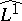). Frequently, the series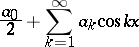(a2)

and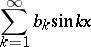(a3)

are investigated separately, since there is a difference in their behaviour, and usually integrability of (a3) requires additional assumptions. Of course, one may also consider trigonometric series in complex form.

There exists no convenient description ofin terms of a given sequence alone. Hence, subspaces ofare studied. In view of the Riemann–Lebesgue lemma (cf. Fourier series)is a subspace of the space of null sequences, while the space of sequences of bounded variation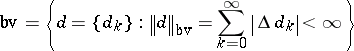is not a subspace of. Here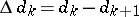. Having a null sequence of bounded variation as its Fourier coefficients, the series (a2) converges for every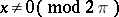, while (a3) converges everywhere.

In 1913, W.H. Young [a20] proved that ifis a convex null sequence, that is,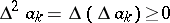for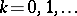, then (a1) is the Fourier series of an integrable function (cf. also Trigonometric series). In 1923, A.N. Kolmogorov [a12] extended this result to the class of quasi-convex sequences, namely, those satisfying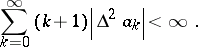Such a sequence is the difference of two convex sequences. In 1956, R.P. Boas generalized all previous results [a6]. Subsequently, more general subspaces ofwere considered:

1) The so-called Boas–Telyakovskii space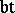(see, e.g., [a16]):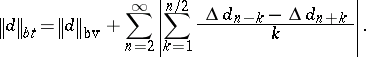2) The Fomin space[a7] for,: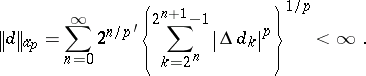3) The Sidon–Telyakovskii space [a17]:4) The Buntinas–Tanovic–Miller spaces (see, e.g., [a2]).

5) The amalgam space [a1], [a3]: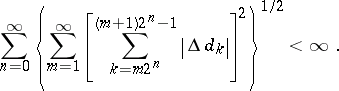A classical way to prove such results is by using Sidon-type inequalities (see, e.g., [a8]), a typical example of which is the one obtained by S. Sidon [a15]: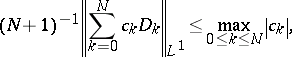whereis the Dirichlet kernel of order.

In [a13] a new approach to these problems was suggested. First, a locally absolutely continuous functiononis considered such that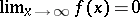(cf. also Absolute continuity) and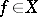, whereis a subspace of the space of functions of bounded variation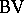and is a generalization of a known space of sequences; e.g., 1)–3) above. Then the asymptotic behaviour of the Fourier transform of a function fromis investigated. Using the following result from [a19] (an earlier version for functions with compact support can be found in [a5]),one obtains even stronger results than those known earlier (for early results, see [a18], [a19]).

Results on integrability of trigonometric series have numerous applications to approximation problems. The Lebesgue constants of linear means of Fourier series can be efficiently estimated in this way (see, e.g., [a16]). For applications to multiplier problems, see [a11] and [a14]. Other integrability conditions (see, e.g., [a4] and [a16]) were surprisingly applied to the approximation of infinitely differentiable functions in [a10] and [a9].

There exist various extensions of integrability conditions for trigonometric series to the multi-dimensional case (see, e.g., [a13]).